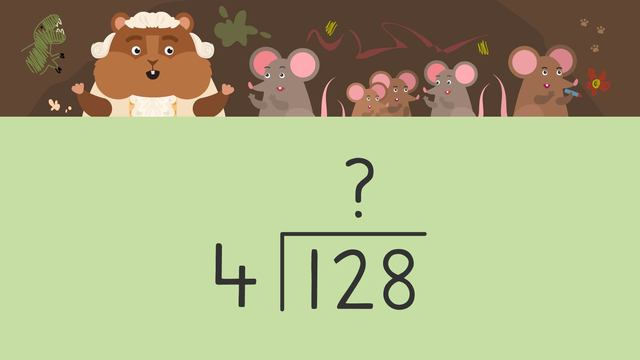# Long Division- Multi digits by 1 digitRating

Ø 5.0 / 2 ratings

The authorsTeam Digital

## Basics on the topicLong Division- Multi digits by 1 digit

### Content

####In this Long Division - Multi-Digit by 1 Digit Video Mr. Squeaks is trying to earn enough money to be able to attend his next adventure: The George Washington, Crossing the Delaware River Reenactment. He decides that he will offer babysitting services to earn the funds he needs. Several families hire Mr. Squeaks and we use the method of long division of multi-digit numbers by 1 digit divisors to calculate the amount of money he makes each time he babysits. Will Mr. Squeaks’ babysitting service pay off in the end?

#### Example of Multi Digit Long Division### TranscriptLong Division- Multi digits by 1 digit

Mr. Squeaks needs funds for his next historical adventure, so he's starting a business to raise money! He starts a babysitting service and boy does it take off! Mr. Squeaks wants to calculate exactly how much he has been making! We can help calculate the earnings per babysitting duty with... "Long Division - Multi-Digit by 1 Digit" In long division, we organize the dividend, divisor, and quotient like THIS. In long division, we find the quotient using these steps... Divide, multiply, subtract, bring down, repeat The Field Family hired Mr. Squeaks to watch their kids three times and paid him three hundred sixty-three dollars. We can divide to calculate how much he made EACH time he babysat. To do this, we will divide three-hundred-sixty-three by three. Put the dividend, "three hundred sixty-three" HERE… And the divisor, three, HERE. First, we divide the hundreds in the dividend, by the divisor. Three goes into three, one time. We write the one ABOVE the hundreds place in the quotient. Next, we multiply this number by the divisor. One times three equals three… and write this answer BELOW the hundreds in the dividend. Then, we subtract. Three minus three equals zero. Now, we bring down the number from the tens place... and REPEAT these steps. THREE goes into six, TWO times… so, we write the two above the tens place and multiply it by the divisor. Three times two equals six. write the six HERE... and subtract. Six minus six equals zero. There is still the number in the ones place to bring down... so, we have to repeat the steps ONE MORE time. Three goes into three one time. One times three equals three… and three minus three equals zero. The quotient is one hundred twenty-one. The Field Family paid Mr. Squeaks one hundred, twenty-one dollars each time he babysat. The Vole's hired Mr. Squeaks to babysit five times and paid him "two hundred forty-five" dollars in all. We can divide two hundred forty-five by five to see how much he earned each time. What is our first step? We need to divide the hundreds place by the divisor. What do you notice when you think about how many times five goes into two? Since five is MORE than two, it can not be divided evenly. This time, we need to look at the first two place values TOGETHER. How many times does five go into twenty-four? There are FOUR groups of five in twenty-four. We write the four above the TENS PLACE in the quotient... and multiply the four to the divisor, five. Four times five is twenty. Where do we write the twenty? We put it UNDER the twenty-four. Now, subtract, twenty-four minus twenty equals four. What is our next step? Bring down the next number. What number do we bring down? Five and now we have forty-five HERE. We need to repeat the steps again. How many times does five go into forty-five? Nine times. What do we multiply the nine by? The divisor, five. What is nine times five? Forty-five Write forty-five here... and subtract leaving zero. What is the quotient? Forty-nine. The Vole's paid Mr. Squeaks forty-nine dollars each time. Remember... long division is a way to organize the calculation of a division problem. To divide we follow these steps: Divide Multiply Subtract Bring down next place value in the dividend. Repeat these steps until all digits in the dividend have been used. Mr. Squeaks has earned enough money to go on his long-awaited adventure. Mr. Squeaks! Mr. Squeaks! Mr. Squeaks, it’s time for you to go on your trip. [Mr. Squeaks closes his eyes again…too exhausted to move.]